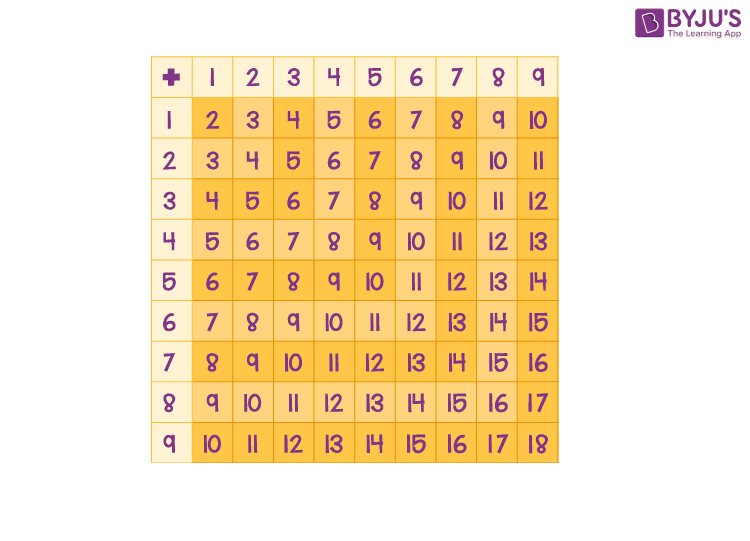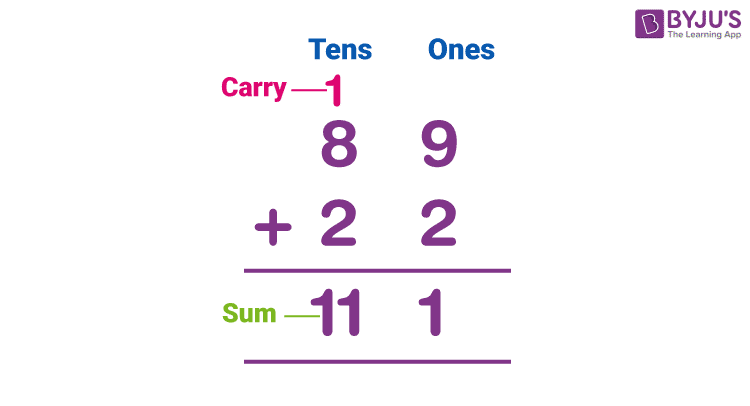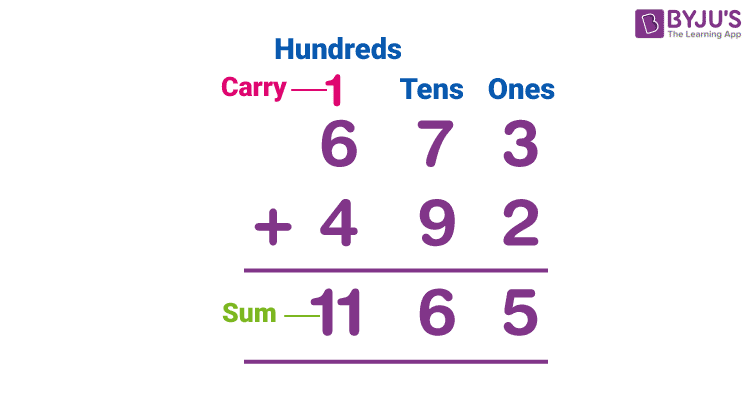# Sum

The term Sum in Maths refers to the result obtained through the addition of numbers, objects or things. We use this term very frequently in our daily existence. For example, when we purchase a certain number of items, such as fruits and vegetables, we ask the seller to add up the total amount that we need to pay for the purchased items. Here, the total amount is called the sum. In this article, you will learn the definition of sum, and how to find the sum of numbers in different situations along with illustrations.

## Sum Meaning

In mathematics, the sum can be defined as the result or answer after adding two or more numbers or terms. Thus, the sum is a way of putting things together. In other words, the sum is the process of bringing two or more numbers together to produce a new result or total.Here, 5 and 7 are the addends and 12 is the sum of 5 and 7.

### Sum Notation

A plus symbol (+) is used when we add the numbers. The sum is the name of the result obtained through addition. We can represent the sum by the symbol ∑ (sigma). Generally, it is used when we have to add an extensive list or a sequence of numbers.

## Sum of Digits

Let’s understand how to find the sum of one-digit numbers, two-digit numbers, three-digit numbers, etc., with detailed explanations here.

### Sum of One-digit Numbers

We can get the sum of 2 one-digit or single-digit numbers such as 1, 2, 3, 4, 5, 6, 7, 8, 9 in different combinations from the below chart.In the above figure, the sum of two equal numbers can be observed diagonally across the plus (+) symbol.

### Sum of Two-digit Numbers

Finding the sum of two-digit numbers sometimes involves carrying out the obtained result. The below steps are useful for finding the sum of numbers with two digits.

Step 1: Write the given numbers in the column by providing enough space between digits for easy understanding.

Step 2: Add the ones place digits together, and transfer the carry (if any). Finally, it gives the sum of numbers that are in the unit place.

Step 3: Add the tens place digits and carry from the previous step (if any) and transfer the carry. It gives the sum of numbers in the tens place.

Step 4:Thus, the digits in the final row represent the sum of given numbers.

The below example shows the summation of two-digit numbers.

Example: The sum of 89 and 22 can be calculated as:Therefore, the sum of 89 and 22 is 111.

In the same way, it is possible to add more than two numbers.

### Sum of Three-Digit Numbers

Now, go through the process of finding the sum of the three-digit numbers given below:

Step 1: Write the given numbers in the column by providing enough space between digits for easy understanding.

Step 2: Add the ones place digits together, and transfer the carry (if any). Finally, it gives the sum of numbers that are in the unit place.

Step 3: Add the tens place digits and carry from the previous step (if any) and transfer the carry. It gives the sum of numbers in the tens place.

Step 4: Add the digits in hundreds place digits, and carry the number from the previous step (if any). Thus, it provides the hundreds or thousands or both (depending on the sum) of the result.

Step 5: Thus, the digits in the final row represent the sum of given numbers.

A similar procedure can be applied to find the sum of large digit numbers. Let’s have a look at the example given below.

Example: Find the sum of 673 and 492.### Sum of First n Natural Numbers

As we know, a sum is the result of summation or the addition of a sequence of numbers. Thus, we can find the sum of the sequence of first n natural numbers.

The first n natural numbers are:

1, 2, 3, 4,…., n

This is an AP with first term a = 1 and last term l = n.

We know that, the sum of n terms of AP when the first and last terms are known is given by:

S = (n/2) (a + l)

= (n/2)(1 + n)

= n(n + 1)/2

Therefore, the sum of the first n natural numbers is given by n(n + 1)/2.

### Sum of Odd numbers formula

The sequence of odd numbers is:

1, 3, 5, 7, 9, 11,…..

This is an AP with the first term a = 1 and the second term a + d = 3.

Common difference = d = 3 – 1 = 2

Sum of the first n odd numbers is:

S = (n/2) [2a + (n – 1)d]

= (n/2) [2(1) + (n – 1)(2)]

= (n/2) [2 + 2n – 2]

= (n/2) 2n

= n2

Therefore, the sum of the odd numbers formula is n2.

### Sum of Even numbers formula

The sequence of even numbers is:

2, 4, 6, 8, 10,…..

This is an AP with the first term a = 2 and the second term a + d = 4.

Common difference = d = 4 – 2 = 2

Sum of the first n odd numbers is:

S = (n/2) [2a + (n – 1)d]

= (n/2) [2(2) + (n – 1)(2)]

= (n/2) [4 + 2n – 2]

= (n/2) [2(n + 1)]

= n(n + 1).

Therefore, the formula for the sum of even numbers is n(n + 1).

### Sum of Squares of n Natural Numbers

The formula for the sum of squares of n natural numbers is given as:

Σn2 = [n(n+1)(2n+1)]/6

This formula is used to find the sum of the squares of the first n positive integers.

### Sum of cubes of n natural numbers

The formula for the sum of cubes of n natural numbers is:

∑n3 = [n(n + 1)/2]2

### Word Problems on Sum

Question 1: In bag A, there are 10 balls and in bag B there are 17 balls. Find the total number of balls.

Solution:

Number of balls in bag A = 10

Number of balls in bag B = 17

Total number of balls = 10 + 17 = 27

Question 2: Gautam has five 2 rupee coins, and Kamal has 10 one rupee coins, whereas Veena has seven 5 rupee coins. So what is the sum of money that Gautam, Kamal and Veena have?

Solution:

According to the given information,

 Person Amount Gautam 5 × Rs. 2 = Rs. 10 Kamal 10 × Rs. 1 = Rs. 10 Veena 7 × Rs. 5 = Rs. 35

Sum of money = Rs. 10 + Rs. 10 + Rs. 35 = Rs. 55

### Practice Problems

1. Find the sum of the first 15 natural numbers.
2. What is the sum of the first 10 even numbers?
3. Find the sum of the squares of the first 20 natural numbers.

## Frequently Asked Questions on Sum

Q1

### Does the sum mean to add?

In mathematics, the term sum refers to the result obtained when we add numbers, objects or things. For example, addends 14 and 6 add up to make a sum of 20.

Q2

### What is the symbol for sum?

The symbol used to denote the sum of a list of numbers is ∑.

Q3

### What is the sum of numbers from 1 to 100?

The sum of numbers from 1 to 100 can be calculated as:
n = 100
Sum of 100 natural numbers = [100(100 + 1)/2] = 50 × 101
= 5050

Q4

### What is the sum of 3 and 5?

The sum of 3 and 5 is 8, i.e. 3 + 5 = 8.

Q5

### What is another word for the sum in math?

In maths, the total amount is obtained when we add two or more numbers, amounts, or items. Here, the sum can also be stated as the total, aggregate or tally.